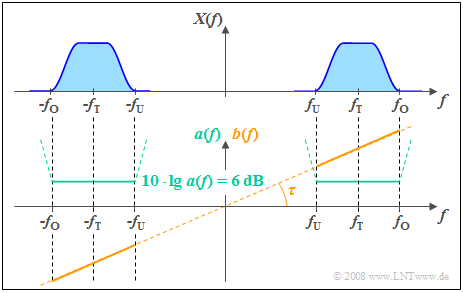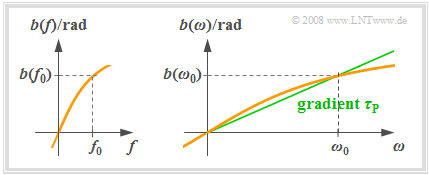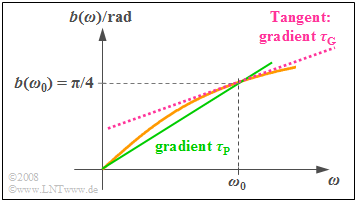# Difference between revisions of "Linear and Time Invariant Systems/Linear Distortions"

## Compilation of important descriptive quantities

Now nonlinear distortions are excluded so that the system is fully described by the frequency response  $H(f)$ .

The generally complex frequency response can also be formulated as follows:

$$H(f) = |H(f)| \cdot {\rm e}^{-{\rm j} \hspace{0.05cm} \cdot \hspace{0.05cm} b(f)} = {\rm e}^{-a(f)}\cdot {\rm e}^{-{\rm j} \hspace{0.05cm} \cdot \hspace{0.05cm} b(f)}.$$

This yields the following descriptive quantities:

• The magnitude  $|H(f)|$  is referred to as  amplitude response  and in logarithmic form as  "attenuation curve":
$$a(f) = - \ln |H(f)|\hspace{0.2cm}{\rm in \hspace{0.1cm}Neper \hspace{0.1cm}(Np) } = - 20 \cdot \lg |H(f)|\hspace{0.2cm}{\rm in \hspace{0.1cm}decibel \hspace{0.1cm}(dB) }.$$
• The  phase response  $b(f)$  specifies the negative angle of  $H(f)$  dependent on  $f$  in the complex plane and with respect to the real axis:
$$b(f) = - {\rm arc} \hspace{0.1cm}H(f) \hspace{0.2cm}{\rm in \hspace{0.1cm}radian \hspace{0.1cm}(rad)}.$$

## Requirements for distortion-free systems

According to the explanations in the chapter  Classification of the Distortions   there is a distortion-free system at hand if and only if all frequency components are uniformly attenuated and delayed:

$$y(t) = \alpha \cdot x(t - \tau).$$

According to the systems theory laws,  the following must thus hold for the frequency response:

$$H(f) = \alpha \cdot {\rm e}^{-{\rm j}\hspace{0.04cm}2 \pi f \tau},$$

or expressed with the functions  $a(f)$  and  $b(f)$:

• The attenuation curve must be constant for all frequencies contained in the input signal:
$$a(f) = - \ln |H(f)| = - \ln \ \alpha = {\rm const.}$$
• The phase response must either be zero in the region of interest  (system with no transit time)  or increase linearly with frequency  $(τ$  indicates the transit time):
$$b(f) = 2 \pi f \tau = {\rm const.} \cdot f.$$

$\text{Definitions:}$  For a distortion-free system, both requirements must be satisfied simultaneously.

Violation of even one of these two conditions results in  linear distortions  which are distinguished according to their cause:

• It comes to  attenuation distortions  if in the frequency range of interest the attenuation curve is not constant:
$$a(f) \ne {\rm const.}$$
• In contrast to this, there are  phase distortions  if the phase function is not linear with respect to $f$ :
$$b(f) \ne {\rm const.} \cdot f.$$

It should be noted that in all  "realizable systems" – in particular, in the  "minimum-phase systems"  described in  Chapter 3  – both forms of distortions usually occur simultaneously.

$\text{Definition:}$  In the time domain, the condition for a  distortion-free system is:

$$h(t) = \alpha \cdot \delta(t - \tau),\hspace{0.4cm}\alpha \ne 0.$$

Moreover,  if  $α = 1$  and  $τ = 0$  hold,  then there is an  ideal transmission system.  In contrast, there are linear distortions whenever

• The impulse response  $h(t)$  is a continuous-time function, or
• the time-dicrete impulse response $h(t)$  is composed of more than one Dirac functions.

$\text{Example 1:}$  The following sketch shows the attenuation curve  $a(f)$  and the phase response  $b(f)$  of a distortion-free system.Requirements for a non-distorting channel:
$f_{\rm U}$:  lower  $($German:  "untere"   ⇒   "U"$)$  range limit,
$f_{\rm O}$:  upper  $($German:  "obere"   ⇒   "O"$)$  range limit,
$f_{\rm T}$:  carrier  $($German:  "Träger"   ⇒   "T"$)$  frequency
• In a range from  $f_{\rm U}$  to  $f_{\rm O}$  around the carrier frequency  $f_{\rm T}$,  in which the signal $x(t)$  has components,  $a(f)$  is constant.
• From the given constant attenuation value  $6 \ \rm dB$  it follows for the amplitude response:  $\vert H(f)\vert = 0.5$.
• The output spectrum  $Y(f)$  is thus half as large in magnitude as the spectral components $X(f)$  of the input signal.
• The phase response  $b(f)$  increases linearly with frequency between $f_{\rm U}$ and $f_{\rm O}$.
• This results in all frequency components being delayed by the same phase delay time  $τ$,  where  $τ$  is fixed by the slope of  $b(f)$ .
• With  $b(f) = 0$  a transit time-free system would arise as a result   ⇒   $τ = 0$.

Furthermore,  the following generally valid properties can be identified from the graph:

• The attenuation curve  $a(f) = a(\hspace{-0.01cm}-\hspace{-0.08cm} f)$  is an even function in  $f$.
• The phase curve  $b(f) = \hspace{0.1cm}–\hspace{-0.01cm} b(\hspace{-0.01cm}-\hspace{-0.01cm}f)$  is an odd function in $f$.

Outside the frequency band occupied by $x(t)$  the  "constant attenuation"  and  "linear phase"  conditions do not need to be satisfied.

It can be seen from the dashed curve of $a(f)$ that even a much higher attenuation is purposeful here because as a consequence the always-existing noise components outside the useful bandwidth – which are not considered in this section – are suppressed better.

## Attenuation distortions

In the following,  we consider the sum of two harmonic oscillations as input signal:

$$x(t) = A_1 \cdot \cos(2 \pi f_1 \cdot t - \varphi_1) + A_2 \cdot \cos(2 \pi f_2 \cdot t - \varphi_2).$$
• If the output signal can be represented in the form
$$y(t) = \alpha_1 \cdot A_1 \cdot \cos(2 \pi f_1 \cdot t - \varphi_1) + \alpha_2 \cdot A_2 \cdot \cos(2 \pi f_2 \cdot t - \varphi_2),$$
and at the same time  $α_1 ≠ α_2$  is valid,  then  exclusively attenuation distortions  are existent since the phase values  $\varphi_1$  and  $\varphi_2$  are not changed by the system.
• The attenuation constants  $α_1$  and  $α_2$  can be determined from the amplitude response  $|H(f)|$ :
$$\alpha_1 = |H(f_1)|,\hspace{0.4cm}\alpha_2 = |H(f_2)|.$$
• If the attenuation curve  $a(f)$  is given in Neper,  then likewise the following holds  $(1 \ \rm dB$ corresponds to $0.1151 \ \rm Np)$:
$$\alpha_1 = {\rm e}^{-{\rm a}(f_1)},\hspace{0.4cm}\alpha_2 = {\rm e}^{-{\rm a}(f_2)}.$$

Please note:   In some character fonts,  "$a$"  and  "$α$"  (alpha)  are difficult to distinguish.

$\text{Example 2:}$  The graph shows the input signal  (blue curve)  which is periodic with  $T_0 = 1\ \rm ms$

$$x(t) = {1\, \rm V} \cdot \cos(2 \pi \cdot {1\, \rm kHz}\cdot t) + {1\, \rm V} \cdot \sin(2 \pi \cdot {2\, \rm kHz}\cdot t)$$

and the signal  $y(t)$  (red curve)  which is attenuation-distorted with  $α_1 = 0.2$,  $α_2 = 0.5$.

Significant effects of these attenuation distortions can be perceived:

• $y(t)$  bears little resemblance to  $x(t)$.
• In contrast, considering  $α_1 = α_2 = α$  the distortion-free signal  $y(t) = α · x(t)$  would be obtained from which the original signal  $x(t)$  could be reconstructed by amplifying it by $1/α$.

We refer here explicitly to the interactive applet  Linear distortions of periodic signals.

## Phase delay time

We consider a system with  $|H(f)| = 1$  such that the following holds for the frequency response:

$$H(f) = {\rm e}^{-{\rm j} \hspace{0.05cm} \cdot \hspace{0.05cm} b(f)}.$$
• The left graph shows an exemplary phase response  $b(f)$.  Such a phase response is always an odd function with respect to the frequency $f$:   $b(\hspace{-0.01cm}-\hspace{-0.08cm}f) = \hspace{0.08cm}-b(f)$.
• On the right, the function  $b(ω)$  is sketched which is dilated by a factor of  $2π$  with respect to  $b(f)$  in the abscissa.

If the harmonic oscillation at the input is

$$x(t) = C \cdot \cos(2 \pi f_0 t - \varphi)\hspace{0.15cm} \circ\!\!-\!\!\!-\!\!\!-\!\!\bullet\, X(f ) = {C}/{2}\cdot {\rm e}^{{\rm j}\hspace{0.05cm} \cdot \hspace{0.05cm} \varphi} \cdot \delta(f + f_0) \hspace{0.01cm} + \hspace{0.01cm}{C}/{2}\cdot {\rm e}^{-{\rm j}\hspace{0.05cm} \cdot \hspace{0.05cm} \varphi} \cdot \delta(f - f_0),$$On the definition of the phase delay time $\tau_{\rm P}$

then the following arises as a result for the spectral function at the output:

$$Y(f ) = {C}/{2}\cdot {\rm e}^{{\rm j}\hspace{0.05cm} \cdot \hspace{0.05cm} \varphi} \cdot {\rm e}^{\hspace{0.05cm}{\rm j}\hspace{0.05cm} \cdot \hspace{0.05cm} b(f_0)} \cdot \delta(f + f_0) \hspace{0.05cm} + \hspace{0.05cm}{C}/{2}\cdot {\rm e}^{-{\rm j}\hspace{0.05cm} \cdot \hspace{0.05cm} \varphi}\cdot {\rm e}^{-{\rm j}\hspace{0.05cm} \cdot \hspace{0.05cm} b(f_0)} \cdot \delta(f - f_0).$$

Thus, the output signal is:

$$y(t) = C \cdot \cos(2 \pi f_0 t - b(f_0) - \varphi).$$

This signal can also be represented in the following form:

$$y(t) = C \cdot \cos(2 \pi f_0 ( t - \tau_{\rm P}(f_0)) - \varphi).$$

$\text{Definition:}$  The  phase delay time  indicates the delay experienced by a harmonic oscillation of frequency  $f_0$  through the system.  For phase-distorting systems, the phase delay time is frequency-dependent:

$$\tau_{\rm P}(f_0) = \frac{b(f_0)}{2\pi f_0} \hspace{0.4cm}{\rm or} \hspace{0.4cm} \tau_{\rm P}(\omega_0) = \frac{b(\omega_0)}{\omega_0}.$$

The following should be noted regarding this definition:

• In the  $b(ω)$  representation,  the phase delay time  $τ_{\rm P}$  can also be determined graphically as the slope of the straight line drawn in green in the above graph.
• In general,  an oscillation with a different frequency will also result in a different phase delay time.  This is the physical background for phase distortions.
• If for a system  $b(ω) = τ_{\rm P} · ω$   or   $b(f) = 2π · τ_{\rm P} · f$  is true,  then all frequencies have the same phase delay time  $τ_{\rm P}$.  Such a system does not cause phase distortions.

We refer here again to the interactive applet  Linear distortions of periodic signals.

## Difference between phase and group delay time

Another important system description quantity is the group delay time which must not be confused with the phase delay time.

$\text{Definition:}$  The  group delay time  is defined as follows:

$$\tau_{\rm G}(\omega_0) = \left[ \frac{ {\rm d}b(\omega)}{ {\rm d}\omega}\right ]_{\omega = \omega_0}.$$
• This quantity is mainly used to describe narrow-band systems.
• It indicates the delay experienced by the envelope of a band-pass system.On the definition of the group delay time $\tau_{\rm G}$

$\text{Example 3:}$  The graph shows the examplary phase function:

$$b(ω) = \arctan (ω/ω_0).$$
• This increases monotonically from zero  $($at   $ω = 0)$  to  $π/2$  $($for   $ω → ∞)$.
• The function value at  $ω = ω_0$  is  $π/4$.

If we set  $ω_0 = 2π · 1 \ \rm kHz$, we obtain for the  phase delay time:

$$\tau_{\rm P}(\omega_0) = \frac{b(\omega_0)}{\omega_0}= \frac{\pi / 4}{2 \pi \cdot{1\, \rm kHz} } = {125\, \rm µ s}.$$

⇒   This quantity corresponds to the slope of the straight line drawn in green in the graph.

In contrast, the lesser slope of the tangent line shown in red denotes the  group delay time:

$$\tau_{\rm G}(\omega_0) = \left[ \frac{ {\rm d}b(\omega)}{ {\rm d}\omega}\right ]_{\omega = \omega_0} = \left[ \frac{1}{\omega_0} \cdot \frac{1}{1 + \left(\omega / \omega_0\right]^2} \right ]_{\omega = \omega_0} = \frac{1}{2\omega_0}= \frac{1}{4 \pi \cdot{1\, \rm kHz} } \approx {80\, \rm µ s}.$$

## Phase distortions

To illustrate this point, we again consider the sum of two harmonic oscillations as input signal:

$$x(t) = A_1 \cdot \cos(2 \pi f_1 \cdot t - \varphi_1) + A_2 \cdot \cos(2 \pi f_2 \cdot t - \varphi_2).$$
• If the output signal for this input signal can be represented in the form
$$y(t) = A_1 \cdot \cos(2 \pi f_1 \cdot (t - \tau_1) - \varphi_1) + A_2 \cdot \cos(2 \pi f_2 \cdot (t - \tau_2) - \varphi_2)$$
and at the same time  $τ_1 ≠ τ_2$ is valid, then   exclusively phase distortions  are existent.
• The two phase delay times  $τ_1 ≠ τ_2$  can be determined from the phase response  (in radian):
$$\tau_1 = \frac{b(f_1)}{2\pi f_1} , \hspace{0.4cm}\tau_2 = \frac{b(f_2)}{2\pi f_2}.$$

$\text{Example 4:}$  The graph shows the periodic signal with period  $T_0$   ⇒   blue curve

$$x(t) = {1\, \rm V} \cdot \cos(2 \pi \cdot {1\, \rm kHz}\cdot t) + {1\, \rm V} \cdot \sin(2 \pi \cdot {2\, \rm kHz}\cdot t)$$

as well as the signal  $y(t)$  which is phase-distorted with the transit times  $τ_1 = 0.7 \ \rm ms$  and  $τ_2 = 0.3 \ \rm ms$  ⇒   red curve.

• The effects of the phase distortions can be clearly seen.
• With   $τ_1 = τ_2 = τ$   the following distortion-free signal would arise as a result:
$$y(t) = x(t - τ).$$

We refer once more to the interactive applet  Linear distortions of periodic signals.

## Equalization methods

This method, which is very important for Communications Engineering,  will only be briefly touched upon here.  More detailed information can be found in the books  Modulation Methods  and  Digital Signal Transmission.

For this brief description, we assume the outlined constellation:

• $S_{\rm V}$  denotes a distorting system, because of  "distortion"  ⇒   German:  "Verzerrung"   ⇒   "V",
• while $S_{\rm E}$  serves for equalization   ⇒   "E".

Regarding this constellation,  the following should be noted:

• If the distortion is nonlinear,  the equalization must also be nonlinear.
• But even with linear distortion,  nonlinear equalization methods are used, e.g.  "Decision Feedback Equalisation"  in digital systems.  The advantage over linear equalization is that there is no increase in noise power.
• If  $S_{\rm V}$  is a linear system with frequency response  $H_{\rm V}(f)$,  then the distortions can be completely eliminated with the  inverse frequency response  $H_{\rm E}(f) = 1/H_{\rm V}(f)$  and  $z(t) = x(t)$  holds.  However,  a prerequisite for this is that the frequency response  $H_{\rm V}(f)$  has "no zeros"  in the spectral range of interest,  otherwise infinity points would be required for  $H_{\rm E}(f)$ .
• For  analog systems,  complete equalization means that  $z(t)$  differs from  $x(t)$  only by the unavoidable noise components and possibly by a transit time.
• For  digital systems,  the criterion for complete equalization is less strict.  It must then only be ensured that the signals  $x(t)$  and  $z(t)$  coincide at the detection times.  In this context,  one deals with  Nyquist systems.